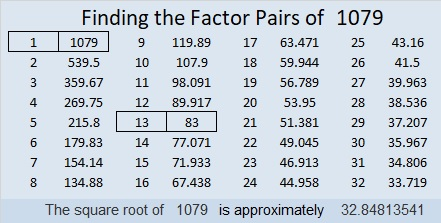# 1079 An Easier Find The Factors Challenge?

This find the factors challenge puzzle might be easier than most of the challenge puzzles are, but it will still give you plenty of reasons to think about what factors you should put where. You need to put all the numbers from 1 to 10 in each of the four bold areas so that those numbers are the factors of the given clues. There is only one solution. Can you find it?Print the puzzles or type the solution in this excel file: 10-factors-1073-1079

Here’s a little about the number 1079:

• 1079 is a composite number.
• Prime factorization: 1079 = 13 × 83
• The exponents in the prime factorization are 1 and 1. Adding one to each and multiplying we get (1 + 1)(1 + 1) = 2 × 2 = 4. Therefore 1079 has exactly 4 factors.
• Factors of 1079: 1, 13, 83, 1079
• Factor pairs: 1079 = 1 × 1079 or 13 × 83
• 1079 has no square factors that allow its square root to be simplified. √1079 ≈ 32.848141079 is the sum of three consecutive prime numbers:
353 + 359 + 367 = 1079

1079 is also the hypotenuse of a Pythagorean triple:
415-996-1079 which is (5-12-13) times 83

This site uses Akismet to reduce spam. Learn how your comment data is processed.